# Multiple Ways to Optimize Rheology for Increased Dispersion, Colloidal and Emulsion Stability

This article looks at ten key points regarding the basic techniques of designing and interpreting rheological tests to improve the stability of a sample. The ten points are mainly applicable to colloidal systems, which have a dispersed phase size of <1mm:

• Emulsions are liquid-in-liquid systems and used in foodstuffs, paints and coatings, pharmaceutical formulations, agrochemicals, cosmetics, personal care and adhesives.
• Sols are solid-in-liquid systems such as pharmaceutical formulations, inks, paints and coatings, food and drink, cosmetics, and personal care.

However, these techniques can also be used to evaluate classical non-colloidal systems such as ceramics, cement and mineral slurries.

Each point emphasizes on one property of the material and examines ways to optimize sample stability.

## Increase the Zero Shear Viscosity of a Material

The viscosity exhibited by a material at a shear rate approaching zero is defined as the zero shear viscosity.

This means the viscosity at rest, which is the required condition for stability analysis, and the material is exposed only to the gravitational force. Higher viscosity at lower shear rates prevents any suspended particles from settling. A rheometer is preferred to determine very low shear rates for identification of the zero shear rate viscosity plateau owing to the involvement of lower velocities and torques (Figure 1).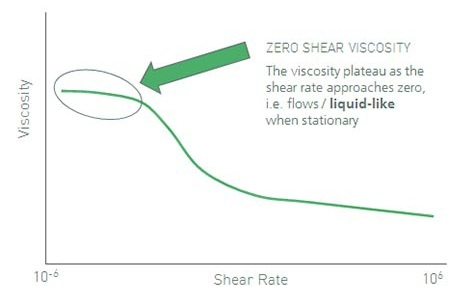Figure 1. The viscosity exhibited by a material at a shear rate inclining to zero is defined as the zero shear viscosity.

## Introduce Yield Stress Type Behavior to the Material

The fact is that high zero shear viscosity provides good stability, and hence the highest zero shear viscosity possible would be ideal, which would be infinity. This means the flow of an infinite resistance below a critical shear stress, which is defined as yield stress (Figure 2). When yield stress is introduced into a material, the material begins to act like a solid at rest, which innately provides high resistance to any suspended material to sedimentation.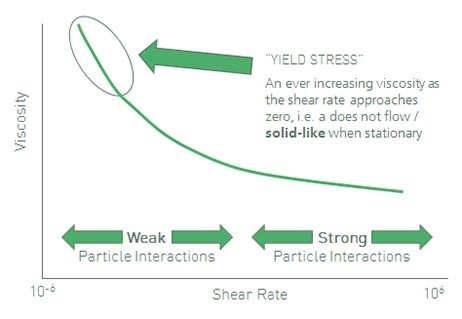Figure 2. An infinite resistance to flow below a critical shear stress is defined as yield stress.

## Increase the Value of Yield Stress

The yield stress indicates a solid-like behavior at rest which can resist settling. The actual yield stress can however have a broad value range, with a higher value signifying more resistance to sedimentation. Therefore, a suspension with a higher yield stress value will have very high stability (Figure 3).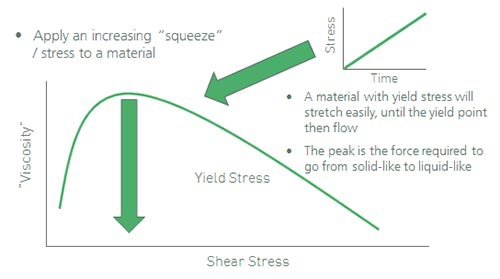Figure 3. A suspension will be more stable with a higher value of yield stress.

## Minimize the Sample’s Thixotropy

Even though stability is known to be a low stress process, many materials are subjected to higher stresses during transportation than they are at rest. The shear thinning nature of typical dispersions makes them to lose viscosity at higher stress conditions. This, in turn, reduces the resistance to sedimentation. The stability of a sample can be increased by shortening the time of maintaining the material to these low viscosities through reduction of the extent of thixotropy (Figure 4).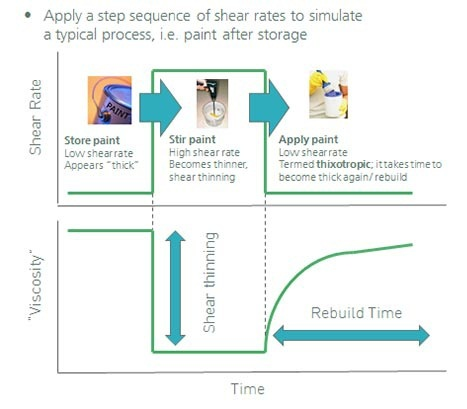Figure 4. This image illustrates a step sequence of shear rates.

## Increase the Cohesive Energy of the sample

The cohesive energy represents the elastic strength of the internal structure of a material and is in correlation with the yield stress. A system will have better stability if the cohesive energy is on the higher side. To calculate the cohesive energy, an amplitude sweep is performed on the material to identify the strain limit of the Linear Viscoelastic Region (LVR). Squaring and multiplying the strain limit value by half the value of the magnitude of the storage modulus in the LVR (Figure 5).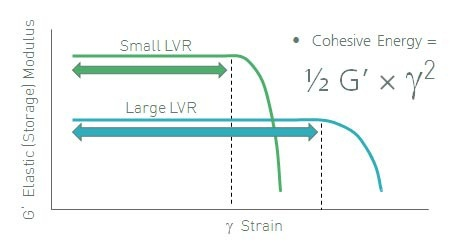Figure 5. The cohesive energy is a measure of the elastic strength of the materials internal structure.

## Optimize the Viscoelastic Character – Part I

To determine the properties of a material over different time scales, viscoelastic spectrum of the material has to be measured using a frequency sweep. Generally, viscoelastic liquids are less stable due to the increase of phase angle under low frequencies. This makes the sample more liquid like. High- phase angles reveal that the material as well as the suspended particles tends to flow and settle when left undisturbed.

A probable solution to this problem is to produce a gel-like structure with a phase angle below 45° and independent of frequency (Figure 6). This specifies a yield stress in the measured frequency range and hence is less likely to behave liquid-like at lower frequencies.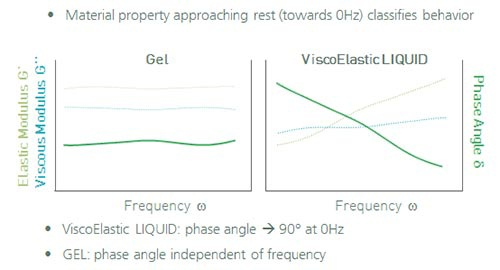Figure 6. Gel with a phase angle independent of frequency and a viscoelastic liquid with a phase angle approaching 90° at 0Hz.

## Optimize the Viscoelastic Character – Part II

Despite the fact that a gel-like sample may display better stability than a viscoelastic liquid, this gel structure may not be good enough to prevent settling for some systems containing heavy or large particles. Hence, a viscoelastic solid-like frequency response might be a solution. A material acts like a solid at low phase angles, thus providing a very stable system (Figure 7).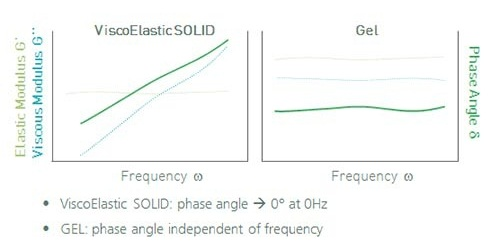Figure 7. Material property approaching rest (towards 0Hz) classifies behavior.

## Increase the Material Resistance to Creep

A creep test helps assessing a material’s flow resistance when exposed to tiny constant forces over prolonged timescales (Figure 8). The creep test can be used to apply a limited force to measure if a material can resist gravitational forces. The smaller the resultant movement, the chances of it being unstable becomes lesser.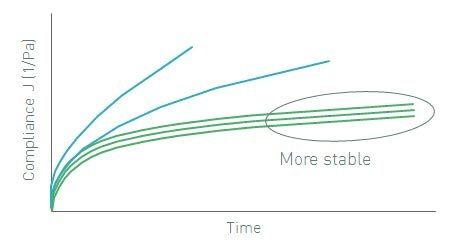Figure 8. A creep test can be used to evaluate a materials resistance to flow under small constant forces.

## Change the Particle Size

Particle-particle interactions have influence over low shear viscosity. Increasing the number of particle-particle interactions can increase the count of particles in a system. For this purpose, the particle size is reduced or particle concentration is increased. Since the effect of gravitational force is significantly less on smaller particles, they show more resistance to sedimentation (Figure 9).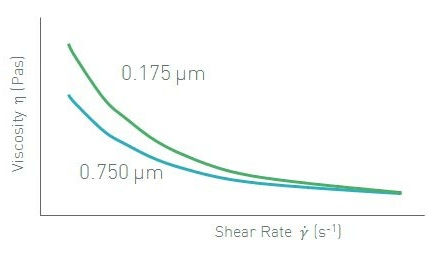Figure 9. Changing the particle size is one method of improving the stability of a system.

## Change the Particle Size Distribution

When the particle concentration cannot be altered, a change in particle size distribution can influence the low shear viscosity. This, in turn, affects the stability of the system. Particles having a wide distribution are likely to pack better when compared to particles having a narrow distribution. This proves that particles with wide distribution have more free space to move, thus enabling easier flow of a sample. This means that the viscosity of the sample is lower. Hence, it is possible to increase the stability of a system by minimizing the polydispersity (Figure 10).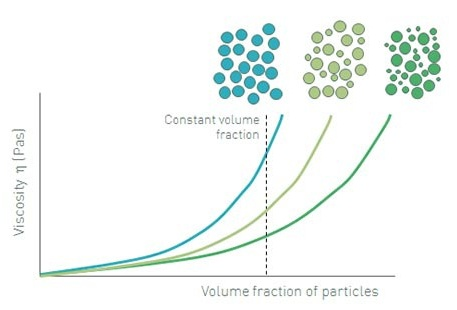Figure 10. Tightening up the particle size distribution will increase the stability of a system.

## Conclusion

The aforementioned methods are useful for rheology optimization in order to increase the stability of emulsions, colloidal systems, and dispersions.This information has been sourced, reviewed and adapted from materials provided by Malvern Panalytical.

## Citations

• APA

Malvern Panalytical. (2019, September 03). Multiple Ways to Optimize Rheology for Increased Dispersion, Colloidal and Emulsion Stability. AZoM. Retrieved on October 21, 2020 from https://www.azom.com/article.aspx?ArticleID=11442.

• MLA

Malvern Panalytical. "Multiple Ways to Optimize Rheology for Increased Dispersion, Colloidal and Emulsion Stability". AZoM. 21 October 2020. <https://www.azom.com/article.aspx?ArticleID=11442>.

• Chicago

Malvern Panalytical. "Multiple Ways to Optimize Rheology for Increased Dispersion, Colloidal and Emulsion Stability". AZoM. https://www.azom.com/article.aspx?ArticleID=11442. (accessed October 21, 2020).

• Harvard

Malvern Panalytical. 2019. Multiple Ways to Optimize Rheology for Increased Dispersion, Colloidal and Emulsion Stability. AZoM, viewed 21 October 2020, https://www.azom.com/article.aspx?ArticleID=11442.